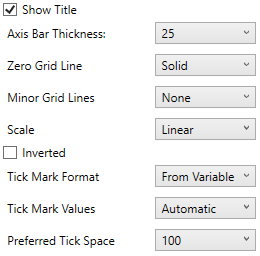Altair® Panopticon

# Numeric Axes

Visualizations supporting numeric axes include the following settings:Setting Description Show Title Displays an Axis Title label. Axis Bar Thickness The margin in pixels for the axis. If set to zero, the axis is removed. Zero Grid Line How a major X-axis grid line is drawn. Allowed values: None Dotted Dashed Solid Minor Grid Line How minor grid lines are drawn across the axis. Allowed values: None Dotted Dashed Solid Bar Scale Determines whether the scale of the axis is Linear, Log 10, or Power. Linear – a change between two values is based on addition e.g., 30, 60, 90, 120, 180, etc. Log 10 – a change between two values is perceived on the basis of the ratio of the two values or based on multiplication. The common base for logarithmic scales is the base 10. For example: log10(x) represents the logarithm of x to the base 10 e.g., 1, 10, 100, 1000, etc. NOTE: Does not support zero or negative values. Power - Works according to the SIGN(MEASURE)*LOG10(MAX(1, ABS(MEASURE))) formula. Works like the Log 10 scale except it can handle negative values and every value between -1 and 1 is set to 0. For example for values between -100 and 100, the axis will be: -100, -10, 0, 10, 100 Inverted Determines whether the Y or Height axis is inverted. Tick Mark Format Set to From Variable to use the format string that is on the current variable displayed in the axis. Set to Metric Prefix to format the Tick labels in the numeric axes using the metric prefixes. Tick Mark Values Set to Automatic for automatic axis ranges or Manual to define specific tick marks. For Automatic: Tick marks (and if enabled Minor Grid Lines) are drawn approximately every n pixels, where n is defined by the Preferred Tick Space list box.   For Manual: The exact position of specific tick marks (and if enabled minor grid lines) can be specified. Additionally a custom label can be specified for each tick mark.   A common usage of Manual tick marks is when displaying yield curves, where the X-axis should display time span: Preferred Tick Space The preferred space in pixels between the minor grid lines across the axis.